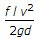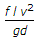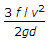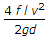# Mechanical Engineering - Hydraulics and Fluid Mechanics - Discussion

3.

According to Darcy's formula, the loss of head due to friction in the pipe is (where f = Darcy's coefficient, l = Length of pipe, v = Velocity of liquid in pipe, and d = Diameter of pipe)

 [A].[B].[C].[D].Answer: Option A

Explanation:

No answer description available for this question.

 Arun B Kumar said: (Nov 26, 2013) Answer D is correct if the "f" denotes friction coefficient. And Answer: A is correct if "f" denotes the friction factor.

 Reuben said: (Apr 9, 2017) I think the answer C is correct.

 Virupaksha said: (Feb 5, 2018) Answer [A] is correct. Because in the question ' f ' is mentioned as Darcy's coefficient/ Darcy's friction factor which is named after the Frenchmen Henry Darcy. It should not be confused with the friction coefficient/ Fanning friction factor, named after American engineer John Fanning. If ' f ' is given as Fanning friction factor then answer D is correct.

 Marghub said: (Feb 12, 2018) I think answer [D] will be correct. According to R.S. Khurmi clearly written f=Darcyes coefficient.

 Mahi said: (Feb 26, 2018) Answer D is correct.

 Farabi said: (Mar 24, 2018) D is the correct option.

 Vinod Koul said: (Apr 4, 2018) D is the correct answer. Ref: R S Khurmi.

 Rasmik said: (Apr 8, 2018) Ans. D is correct. Ref: R S Khurmi.

 Bittu said: (Apr 17, 2018) No, D is the correct option.

 Sagar Jadav said: (May 5, 2018) D is the correct option.

 Gopal Dey said: (May 22, 2018) According to me, D is the correct answer.

 Hardeep said: (Aug 18, 2018) A is correct. Here f is friction factor.

 Sourabh said: (Aug 23, 2018) I think option D is the correct answer because where the diameter is considered. While area consider then it will be option A.

 Srikanth said: (Sep 13, 2018) D is the correct answer.

 Shivaprasad said: (Sep 19, 2018) The correct answer is option D. Ref : R S Kurmi.

 Shivaprasad said: (Sep 19, 2018) The Correct answer is D.

 Sameer said: (Feb 17, 2019) D is the correct option.

 Salahuddin said: (Mar 31, 2019) A is the correct one. Darcy friction factor is four times fanning friction factor.

 Dharmendrasinh said: (Mar 31, 2019) I think D is the correct answer.

 Praveen Tiwari said: (Jul 20, 2019) A is right because friction factor is 4times greater than the coefficient of friction and here in question coefficient of friction given so A is right.

 Kishore Raj said: (Aug 11, 2019) Darcy friction factor = flv^2/2gd. Fanning factor = 4flv^2/2gd.

 Bhanu said: (Sep 5, 2019) 4flv^2/2gd is the correct answer.

 Vin said: (Oct 1, 2019) Both A and D are correct as f in both the cases known as Darcy coefficient or coefficient of friction. And both the formula introduced by Darcy only.

 Siraj (Nmit) said: (Jun 30, 2020) The correct one is D. 4flv^2/2gd According to Darcys or Weisbach equation on major energy loss.

 Asif said: (Jul 7, 2020) D is the correct answer. I too agree.

 Kajal Patil said: (Aug 14, 2020) Answer D is correct. Because they clearly mentioned f is Darcy's coefficient. If they metion f' as a friction factor, then only Answer A will be correct. Because. f' = 4f.

 Sqasi said: (Sep 9, 2020) f' to be 4f only if the flow is turbulent For laminar flow f'=f.

 Yash Agarwal said: (Sep 24, 2020) The right Answer is D.

 Sahil Chavda said: (Sep 25, 2020) The right Answer is D.

 Vinay Bel said: (Oct 21, 2020) The right Answer is D.

 Jaysukh Viramgami said: (May 7, 2021) The correct answer is option D.

 Rajat said: (Jun 7, 2021) Agree, D is the correct answer.

 Rajat said: (Jun 14, 2021) Agree, D is the correct answer.

#### Post your comments here:

Name *:

Email   : (optional)

» Your comments will be displayed only after manual approval.

#### Current Affairs 2021

Interview Questions and Answers